# Application examples for the Markovchart R package

#### 2022-04-09

Control charts originate from industrial statistics, but are constantly seeing new areas of application, for example in health care (Thor et al. 2007, suman2018control). This paper is about the Markovchart package, an R implementation of generalised Markov chain-based control charts with health care applications in mind and with a focus on cost-effectiveness. The methods are based on Zempléni et al. (2004), Dobi and Zempléni (2019a), Dobi and Zempléni (2019b).

This vignette highlights the flexibility of the methods through the modelling of two different disease progression and treatment scenarios, namely for monitoring low-density lipoprotein (LDL) and blood sugar (glycated haemoglobin - HbA1c) levels. The vignette focuses on the practical application of the package’s functions from the viewpoint of a user in the field of biostatistics or health care. For the mathematical background and programming details consult the above-cited papers and the functions’ help pages respectively. For cost calculations, the 2021 March 21 EUR-HUF exchange rate was used (1 EUR = 369.05 HUF).

Note that the code used will often limit the parallelisation of the algorithms for the sake of not to over-burden the system of the user. However, given a powerful hardware setup the package can run much faster.

# Low-density lipoprotein analysis

In the following paragraphs we show a real life healthcare example for LDL monitoring. Two approaches will be presented: one with and one without taking the standard deviation into account. The aim is to minimise the healthcare burden generated by patients with high cardiovascular (CV) event risk. The model is built upon the relationship between the low-density lipoprotein level and the risk of CV events, thus the LDL level is the process of interest (Boekholdt et al. 2012).

Parameters were estimated from several sources. The table below gives information about the meaning of the parameter values in the healthcare setting and shows the source of the parameter estimations.
Parameter value Meaning Parameter source
3 mmol/l Target value Set according to the European guideline for patients at risk
0.1 mmol/l Process standard deviation Estimated using real life data from Hungary, namely registry data through Healthware Consulting Ltd.
0.8/3 Expected shift size, 0.8 increase in LDL per year on average Estimated with the help of a health professional
1/120 Expected number of shifts in a day, 3 shifts per year on average Estimated with the help of a health professional
0.027, 1.15 Parameters of the repair size beta distribution Estimated using an international study which included Hungary
0.1, 30 Parameters of the sampling probability logistic function Patient non-compliance in LDL controlling medicine is quite high, and this is represented through the parametrisation of the logistic function
5.01 EUR Sampling cost Estimated using the LDL testing cost and visit cost in Hungary
4.60 EUR Shift-proportional daily out-of-control (OOC) cost Estimated using real world data of cardiovascular event costs from Hungary
9.97 EUR Base repair cost Estimated using the simvastatin therapy costs in Hungary
7.48 EUR Shift-proportional repair cost Estimated using the simvastatin therapy costs in Hungary

It is very difficult to give a good estimate for the type and parameters of a distribution that properly models the non-compliance, thus the results here can at best be regarded as close approximations to a real life situation. This is not a limiting factor, as patients themselves can have vast differences in their behaviour, so evaluation of different scenarios are often required, and will also be presented here. Since high LDL levels rarely produce noticeable symptoms, the sampling probability only depends on the time between samplings (Institute for Quality and Efficiency in Health Care 2017). Thus, the sampling probability was modelled by the logistic function and not by the beta distribution (see the help page of the Markovstat function for more details). It is important to note that the proportional costs increase according to a Taguchi-type (squared) loss function, thus huge, expenses can be generated if the patient’s health is highly out-of-control.

## Optimisation using only the cost expectation

The LDL aggregated dataset is included in the package with all of the above-mentioned parameter estimations. For cost estimation and optimisation purposes we need to use two function: Markovstat for stationary distribution estimation and Markovchart for cost calculation. The code below feeds the proper parameters to these functions. Notice that exponential shift size distribution, random repair and sampling is used and Markovchart is set for optimisation.

data("LDL")

stat_LDL_cost <- Markovstat(
shiftfun = 'exp', h = 50, k = 0.15,
sigma = LDL$standard_deviation, s = LDL$num_exp_daily_shifts,
delta = LDL$expected_shift_size, RanRep = TRUE, alpha = LDL$rep_size_first, beta = LDL$rep_size_second, RanSam = TRUE, q = LDL$samp_prob_first, z = LDL$samp_prob_second, Vd = 50, V = 3) #Defining parallel_opt parallel settings. #parallel_opt can also be left empty to be defined automatically by the function. num_workers <- min(c(detectCores(),2)) parall <- list(cl=makeCluster(num_workers), forward=FALSE, loginfo=TRUE) res_LDL_cost <- Markovchart( statdist = stat_LDL_cost, OPTIM=TRUE, p = 1, cs = LDL$sampling_cost,
cf = LDL$base_rep_cost, coparams = c(0,LDL$OOC_cost),
crparams = c(LDL$base_rep_cost,LDL$prop_rep_cost),
parallel_opt = parall)
res_LDL_cost
#> $Results #> G-value Expected cost Total cost sd Cost sd due to process var. #> 1 0.4258544 0.4258544 0.5041891 0.5041891 #> 2nd process moment 3rd process moment 4th process moment #> 1 0.4355586 3.703977 77.98069 #> #>$Subcosts
#>   Sampling cost Repair cost OOC cost
#> 1    0.08998974  0.08023143 0.262028
#>
#> $Parameters #> Time between samplings (h) Critical value (k) #> 1 55.70492 0.1583099 The optimal parameters for the case when the cost standard deviation was not taken into account were 55.7 days and 0.158 mmol/l for the time between samplings and the critical increase in the LDL level from the guideline value respectively. These parameters entailed an average daily cost of 0.43€ and standard deviation of 0.5€. This result is interesting, because according to the optimisation we should use a somewhat higher critical value than the one in the guideline – 0 mmol/l critical increase would be the 3 mmol/l value in the guideline. At the same time patients should be monitored more often than usual as times of the LDL measurements are usually several months or years apart. However, this is a strictly cost effective viewpoint which could be overwritten by a health professional. Nonetheless, the results provide a possible new approach to the therapeutic regime for controlling LDL level. Often, it is good to look at the interaction between the parameters and the resulting average cost, especially in situations where the optimal parameters cannot be used because of real life reasons. parall <- list(cl=makeCluster(num_workers), forward=FALSE, loginfo=TRUE) res_LDL_cost_grid <- Markovchart( statdist = stat_LDL_cost, h=seq(50,75,2.5), k=seq(0.05,0.25,0.02), p = 1, cs = LDL$sampling_cost,
cf = LDL$base_rep_cost, coparams = c(0,LDL$OOC_cost),
crparams= c(LDL$base_rep_cost,LDL$prop_rep_cost),
parallel_opt = parall)
plot(res_LDL_cost_grid,
y = 'Expected \ndaily cost',
mid = '#ff9494',
high = '#800000',
xlab = 'Days between samplings',
ylab = 'Critical LDL increase') +
geom_point(aes(x = res_LDL_cost$Parameters[], y = res_LDL_cost$Parameters[]))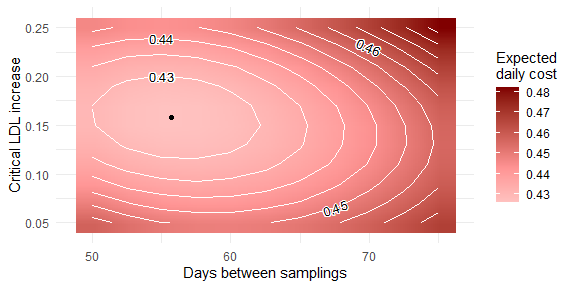The figure shows the expected cost as function of the time between samplings and the critical value.

The heat map shows the average cost as the function of the different parameter values. The dot in the lightest area of Figure $$\ref{expectedcost}$$ corresponds to the optimal cost. Any other point entails a higher average cost. It can be seen that too low or high critical values will both increase the average daily cost. Note that the change in time between samplings entails relatively low change in the critical LDL increase: even if the time between control visits is changed the critical value should stay around 0.12 - 0.18 mmol/l.

## Optimisation using cost expectation and cost standard deviation

The code below is largely the same as before, but notice that p=0.9 instead of p=1, thus prompting the 10% use of the standard deviation during the optimisation process.

stat_LDL_cost <- Markovstat(
shiftfun = 'exp', h = 50, k = 0.15,
sigma = LDL$standard_deviation, s = LDL$num_exp_daily_shifts,
delta = LDL$expected_shift_size, RanRep = TRUE, alpha = LDL$rep_size_first, beta = LDL$rep_size_second, RanSam=TRUE, q = LDL$samp_prob_first, z = LDL$samp_prob_second, Vd = 50, V = 3) parall <- list(cl=makeCluster(num_workers), forward=FALSE, loginfo=TRUE) res_LDL_G <- Markovchart( statdist = stat_LDL_cost, OPTIM=TRUE, p = 0.9, cs = LDL$sampling_cost,
cf = LDL$base_rep_cost, coparams = c(0,LDL$OOC_cost),
crparams= c(LDL$base_rep_cost,LDL$prop_rep_cost),
parallel_opt = parall)
res_LDL_G
#> $Results #> G-value Expected cost Total cost sd Cost sd due to process var. #> 1 0.4266033 0.4323714 0.3746903 0.3746903 #> 2nd process moment 3rd process moment 4th process moment #> 1 0.3273378 2.038361 42.26514 #> #>$Subcosts
#>   Sampling cost Repair cost  OOC cost
#> 1    0.07838653  0.08099193 0.2755366
#>
#> $Parameters #> Time between samplings (h) Critical value (k) #> 1 63.95067 0.145161 In this part, the cost standard deviation is also taken into account as p=0.9, thus the weight of the standard deviation in the calculation of $$G$$ is 0.1. The optimal parameters found by our approach were 63.95 days and 0.145 mmol/l for the time between samplings and critical increase in the LDL level respectively. These parameters entailed an average daily cost of 0.43€ and standard deviation of 0.37€. The inclusion of the cost standard deviation into the model has somewhat increased the time between control visits and decreased the critical value. The expected cost increased, while the cost standard deviation has moderately decreased. The figure below shows the previous heat map with non-compliance included in the model. parall <- list(cl=makeCluster(num_workers), forward=FALSE, loginfo=TRUE) res_LDL_G_grid <- Markovchart( statdist = stat_LDL_cost, h=seq(50,75,2.5), k=seq(0.05,0.25,0.02), p = 0.9, cs = LDL$sampling_cost,
cf = LDL$base_rep_cost, coparams = c(0,LDL$OOC_cost),
crparams= c(LDL$base_rep_cost,LDL$prop_rep_cost),
parallel_opt = parall)
plot(res_LDL_G_grid,
y = 'Expected \ndaily cost',
mid = '#ff9494',
high = '#800000',
xlab = 'Days between samplings',
ylab = 'Critical LDL increase',
nbreaks = 14) +
geom_point(aes(x = res_LDL_G$Parameters[], y = res_LDL_G$Parameters[]))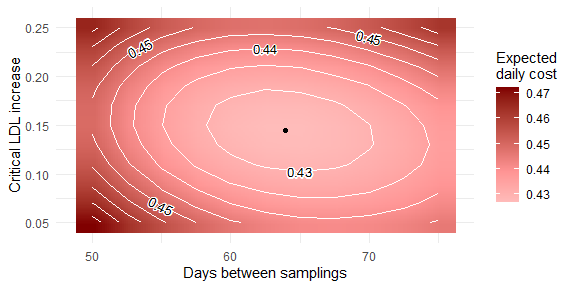The figure shows $$G$$ value (with p=0.9) as function of the time between samplings and the critical value. It can be seen that the elliptical shape of the heat map has not changed: the change in the time between control visits still does not entail great change in the critical value.

## Sensitivity Analysis

As there were uncertainties about the estimation of several parameters, it is important to assess the effect of different parameter setups. The results for different out-of-control costs are plotted for both approaches. The results can be seen in the figure below. The code runs the optimisation repeatedly to collect the necessary data and then gathers the results into a ggplot-compatible data format.

results <- NULL
statds  <- NULL
for (i in 3:10)
{
for (j in c(0.9,1))
{
res_LDL_optim <- Markovchart(
statdist = stat_LDL_cost,
OPTIM = TRUE,
p = j,
cs = LDL$sampling_cost, cf = LDL$base_rep_cost,
coparams = c(0,i),
crparams= c(LDL$base_rep_cost,LDL$prop_rep_cost),
parallel_opt = parall)

results <- rbind(results,c(j,i,unlist(c(res_LDL_optim$Results[c(2,3)],res_LDL_optim$Parameters))))
statds  <- rbind(statds,res_LDL_optim$Stationary_distribution) } } results <- resultsbck <- as.data.frame(results) colnames(results) <- c("p","co","EC","SDC","h","k") results$p           <-  as.character(results$p) results <- cbind(results[,1:2],melt(results[,3:6])) results$variable    <-  as.character(results$variable) results$variable[results$variable=="h"] <- "Days between samplings" results$variable[results$variable=="k"] <- "Critical LDL increase" results$variable[results$variable=="EC"] <- "Expected cost" results$variable[results$variable=="SDC"] <- "Cost standard deviation" results$variable = factor(results$variable, levels=c("Critical LDL increase","Days between samplings","Expected cost","Cost standard deviation")) results$min <-  NA

results$min[results$variable=="Days between samplings"] <- min(results$value[results$variable=="Days between samplings"])
results$max[results$variable=="Days between samplings"] <- max(results$value[results$variable=="Days between samplings"])
results$min[results$variable=="Critical LDL increase"]  <- min(results$value[results$variable=="Critical LDL increase"])
results$max[results$variable=="Critical LDL increase"]  <- max(results$value[results$variable=="Critical LDL increase"])
results$min[results$variable=="Expected cost"]  <- min(results$value[results$variable=="Expected cost"])
results$max[results$variable=="Expected cost"]  <- max(results$value[results$variable=="Expected cost"])
results$min[results$variable=="Cost standard deviation"]    <- min(results$value[results$variable=="Cost standard deviation"])
results$max[results$variable=="Cost standard deviation"]    <- max(results$value[results$variable=="Cost standard deviation"])

app_LDL_params_plot <- ggplot(results, aes(x=co, y=value, group=p)) +
geom_line(aes(colour=p),size = 1.1) +
facet_wrap(~variable, labeller = label_bquote(rows=.(variable)), scales="free_y", nrow=2) +
scale_colour_manual(expression(italic(p)),values=c("black","#800000")) +
geom_blank(aes(y = min)) +
geom_blank(aes(y = max)) +
xlab("Out-of-control cost") +
ylab("Value") +
theme_bw() +
theme(strip.text.x = element_text(size = 11),
strip.text.y = element_text(size = 11,angle = 0), legend.position="top")

app_LDL_params_plot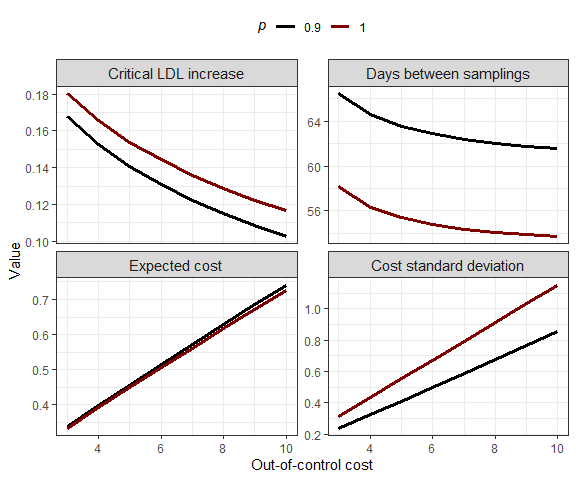The figure shows parameters, average total cost and cost standard deviation as function of the out-of-control cost. The critical value and time between samplings decrease and the average cost and cost standard deviation increase with higher out-of-control costs. Just as on the heat maps, one can observe here, that if the cost standard deviation is taken into account in the optimisation, then the critical value should be lowered and the time between samplings increased. It can be observed that a substantial decrease can be achieved in the cost standard deviation while the cost expectation barely changes.

# Glycated haemoglobin

In this section we will apply the Markovchart package to a randomised real-world data of diabetic patients. The package provides this patient-level pseudonymised and randomised data as the diabetes dataset. The help page of the dataset provides code for data extraction that is useful for control chart applications. This code is skipped here, as it is technical and can be vastly different between applications and datasets. Nonetheless, the reader is invited to study the help page if interested, but the analysis below is based on the already aggregated, useful information gathered from the patient-level data.

## Patient data

The analysis is based on a month-aggregated time series data of diabetic patients from Hungary which was gathered from the period of 2007 September - 2017 September. The data came from two sources: the National Health Insurance Fund of Hungary and the South-Pest Central Hospital. The first source provided information about diagnoses, treatments, health care event and related costs while the latter provided laboratory data regarding blood sugar level. Patients with International Classification of Diseases (ICD) codes (diagnosis) of E10, E11 and E14, and at least one blood sugar measurement were included initially. Only the data of patients with at least one E11 (type II diabetes) diagnosis in the study period was kept. An additional homogenising filter was the requirement of age above 40 at the time of the first diagnosis. Disease progression and therapy effectiveness estimation required at least two blood sugar (HbA1c) measurements with simultaneous therapy data. A total of 4434 patients satisfied all conditions out of which 2151 had at least two HbA1c measurements.

The example study focused on two therapy types: insulin analogues (artificial insulins) and glucagon-like peptides (GLP, promotes insulin secretion). Of course there are more treatment types, the database also lists oral antidiabetics (OAD) and human insulins, but we chose to make the analysis simpler by focusing on GLP and analogue therapies. For the sake of comparison the therapies are grouped in this way: the first group is insulin analogues with possible parallel OAD therapies but human insulin and GLP excluded. The second group is GLP therapies with possible parallel OAD and insulin analogue therapies but human insulin excluded. Essentially we are comparing the effect and cost of insulin analogues with the effect and cost of additional GLP therapies.

The monitored characteristic of the control chart is the blood sugar level, namely the HbA1c level. This is the glycated haemoglobin measured in percent and is slow-changing. The medical aspects of the disease, therapies and blood sugar measurement will not be discussed further as these fall outside of the scope of this example.

The data contains sensitive, patient level information, thus we would only able to show figures, statistics and aggregated data here. However, we pseudonymised and randomised the data in a way that creates fake data but still retains most of the important characteristics and the connections between variables. Namely, random numbers were added (or subtracted) to the date of sampling, the number of sampling per month, costs, HbA1c measurements (both average and standard deviation) and the age of the patient. Furthermore, a subsample was taken from the available patient sample to not even keep the order of patients. The otherwise often very complicated therapeutic regimens were simplified into GLP and analogue categories. This simplification sometimes meant the overwriting of the true therapy used. This is meant to further complicate the identification of patient by e.g., therapeutic pattern. The number of sample elements in the final, randomized dataset can be seen in the table below:

Patient group Total Analogue GLP
Total (all have E11 and are over 40) 800 630 170
E11 ICD 492 272 99

## Parameter estimation

This section provides succinct information about parameter estimation and thus the disease, therapies and the related costs. The aim of the application is to show as many aspects of the Markovchart package to an R user as possible. Due to this, some estimates come from simplified calculations. During industrial or academic applications a group of data scientists and healthcare professionals could provide more accurate estimates, but the work of such a research group is out of the scope of this paper.

Parameters related to disease progression, namely the expected number of shifts per unit time (days) (inverse of shift intensity argument s in the Markovchart function) and the expected shift size delta (delta argument in the Markovchart function) were estimated using the time series data of HbA1c measurements. The delta estimate was calculated from a filtered dataset where we required a HbA1c change larger than $$2\sigma$$ (process standard deviation) and more than 90 but less than 184 days between samplings. The former was necessary to find actual shifts and not just random fluctuations and the latter to try to estimate the size of one shift and not the size of multiple stacked shifts. The expected shift size was delta=1.16. The restriction during estimation of the expected time between samplings was less strict: it was required that the samplings should be at maximum one year (<367 days to account for leap years) from each other. The time between shifts were then gathered from this filtered database and averaged. The shift intensity was calculated by taking the reciprocal of this average and was s=0.0045.

Measurement error (the process standard deviation, sigma argument in the Markovchart function) was estimated using lucky anomalies: the HbA1c level should not be measured more frequently than three months, because the measured values should barely change, and, in relation to this, only 4 measurements are supported per year by the National Health Insurance Fund of Hungary. Nonetheless there were 221 cases in the original data set where HbA1c measurement occurred more than once per month. This number was boosted in the randomised dataset to 692. This data essentially provides information about the measurement error as the actual HbA1c level should change only mildly within such a short time frame. Our estimate with this simple method was sigma = 0.34. It is difficult to compare this result with existing literature due to different methodologies but our estimate is close to the one calculated by Phillipov and Phillips (2001) (even on the randomised data).

Therapy effectiveness was estimated using the definition of effectiveness in the Markov chain-based control charts: the proportion of distance from the target value after treatment compared to the distance before. The target value was set to be 4% HbA1c level, which is the lowest healthy level (Wang 2017). Setting it to a higher value would exclude data, as the target value is the lowest considered. When estimating therapy effectiveness, after the initial filtering of the data, we also restricted HbA1c data to cases where the initial value (after which improvement was seen) was 6%. This was because we wanted to see effect of the therapies only in cases where there is some notable deviation from the healthy state. Improvement was defined as $$>2\sigma$$ (sigma argument in the Markovchart function). Again, values lower than this threshold were considered stagnation (which could also be caused by an effective therapy followed by a degradation, thus making it unreliable) and were not included in the effectiveness estimation. To minimise autocorrelation and the effect of degradation parallel to therapies, only samplings where where more than 90, but less than 184 days elapsed between the two were considered. Parameters of the repair size beta distributions were estimated from the mean and the variance of the ratios of consecutive HbA1c values between samplings. The estimated distribution for analogue therapies was $$beta(2.63,2.05)$$ and for the GLP therapies $$beta(3.85, 3.11)$$ The estimated beta distributions for both therapies can be seen in

therap_plot <-  ggplot(data.frame(x = c(0,1)), aes(x)) +
stat_function(fun = dbeta, aes(colour = ' Analogue', linetype =' Analogue'), size = 1, args = list(shape1 = unlist(ANALOGUE), shape2 = unlist(ANALOGUE))) +
stat_function(fun = dbeta, aes(colour = 'GLP', linetype = 'GLP'), size = 1, args = list(shape1 = unlist(GLP), shape2 = unlist(GLP)), alpha = 0.9) +
scale_colour_manual('Therapy type', values = c('black', '#800000')) +
scale_linetype_manual('Therapy type', values = c('solid', 'dashed')) +
xlab('Therapy effectiveness \n(HbA1c level after therapy divided by the level before)') +
ylab('Beta distribution density') +
theme_bw() +
theme(plot.title = element_text(hjust = 0.5, size = 11), legend.justification = c(1, 0),
legend.position = c(0.20, 0.63), legend.title = element_blank(), legend.margin = margin(t = 0, r = 0.1, b = 0, l = 0, unit = 'cm'),
legend.key = element_rect(fill = 'white', colour = 'white'))
therap_plot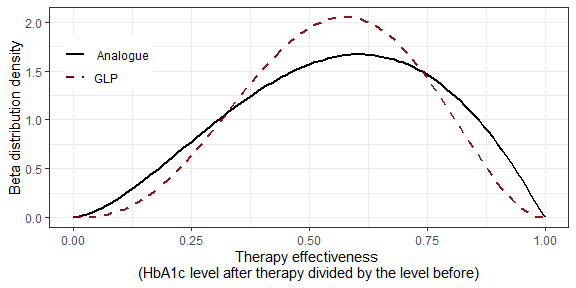Therapy effectiveness comparison using the estimated beta distributions.

It can be seen that the GLP therapy is marginally better based on this dataset than the analogue therapy (judging by the mode). The effectiveness of analogue therapies have a somewhat greater variance. Of course, this method does not take into account the HbA1c level itself, only the change (as a proportion).

Random sampling (i.e., patient non-compliance) was not taken into account as our data did not provide information about this. Nonetheless a sensitivity analysis could be conducted to see the effect of different hypothesised compliance levels, but we have not pursued this idea here.

For daily therapy cost estimation (on top of the initial filtering) we also restricted the data to cases where HbA1c levels were less than or equal to 10%. We observed that cost data as a function of HbA1c level becomes unreliable above this value. This may be due to several factors, one being that patients in highly deteriorated states might have other illnesses (comorbidities) and complications. HbA1c values in between-sampling time periods were imputed using linear interpolation. This was necessary, as therapies were ongoing even between samplings. To be able to estimate cost variances by blood sugar level, the HbA1c level was discretised into 150 categories, as this number was proven to be a good compromise between adequate number of sample elements per category and fineness of the categorisation The relationship between the therapy costs, costs variances and the HbA1c level was then estimated using (non-)linear least squares. This was accomplished with the R function nls. GLP therapy and complication (i.e., OOC) costs in relation of the HbA1c level were estimated and calculated using the default linear cost and cost variance functions, (for more information, see the Markovchart function’s help page). However, the analogue therapy cost and cost variance estimation used a function of the form $c_{r}(v) = c_{rb} + \frac{c_{rs_1}}{v + c_{rs_2}},$ where $$v$$ is the distance from the target value, $$c_{rb}$$ is the base repair cost and $$c_{rs_1}$$, $$c_{rs_2}$$ are the parameters of the scaling repair cost. The resulting functions were the following (everything is given in euro): \begin{align*} c_{analogue}(v) & = 3.3 + \frac{-12.22}{v + 9.37}, \ c_{GLP}(v) = 1.69 + 0.07v, \\ \varsigma_{analogue}(v) & = 7.09 + \frac{-3.32}{v + 1.08}, \ \varsigma_{GLP}(v) = 7 - 0.2v, \\ \end{align*} where $$v$$ is the HbA1c level.

The same restrictions, discretisation and nls function was used when estimating the relationship between the OOC (health care event) cost, cost variance and HbA1c level. Additionally, as there may be a lag between a deteriorated patient state and the resulting health care event, a 6-month cumulative cost was calculated. The resulting functions were the following: \begin{align*} c_{o}(v) & = 0.62 + 0.0062{\cal A}_{h}^2(v), \\ \varsigma_{o}(v) & = 5.12 + 0.13{\cal A}_{h}^2(v), \\ \end{align*} where $$v$$ again is the HbA1c level and $${\cal A}_{h}^2(v)$$ is the expected total squared distance from this starting value on the condition that the sampling interval is $$h$$. Of course, the time between samplings, $$h$$, may not be 6 months in every case, but this was deemed to be a good compromise as there was not enough data to to take $$h$$ into account too during the estimation.

The resulting lines fitted to the data together with standard deviation bands can be seen in the figure below.

cost_plot <- ggplot(data = cost_plots, aes(x = HbA1c, y = Therapy cost)) +
geom_ribbon(aes(x = HbA1c, ymin = low, ymax = high, fill = '±SD'), alpha = 0.4) +
geom_line(aes(x = HbA1c, y = Therapy cost, col = 'Fitted line'), size = 1) +
facet_wrap(Data ~ . ,labeller = label_bquote(rows = .(Data)), ncol = 3) +
scale_color_manual(name = '', values = c('Fitted line' = '#800000')) +
scale_fill_manual(name = '', values = c('±SD' = 'grey70')) +
ylab('Daily cost in EUR') +
theme_bw() +
guides(color = guide_legend(order = 1),
fill = guide_legend(order = 2)) +
theme(legend.position = 'top')

cost_plot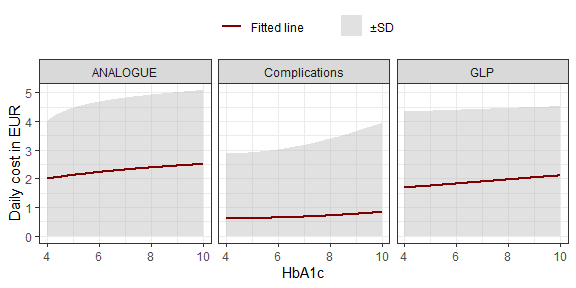Therapy and medical complication costs (€).

It can be seen that analogue and GLP therapies have similar costs, while compared to these, the OOC costs (especially for higher HbA1c levels) are considerably lower. This is expected as diabetes requires constant treatment while complications may or may not occur. Also, it is understandable to keep patients relatively healthy (thus complication-free) even at a high treatment cost.

There are some more parameters that are worth mentioning: for model fit time between samplings and the critical value was also estimated from the data. h was the mean time between samplings (206.22 days), calculated from the randomised patient data. k was determined according to the medical guidelines (7%, see Ton et al. (2013)). constantr=TRUE and ooc_rep=1 were used, as the therapy is constant and occurs even if there is no alarm (alarm states still play an important role as HbA1c reduction can only be initiated by an alarm).

## Stationary distribution and cost estimation with the Markovchart function

Before we can feed the estimated parameters to the Markovchart function, we have to define the above-mentioned custom cost and cost variance functions in R. Afterwards we can run the Markovchart function for both the analogue and the GLP therapy group.

crfun_ANALOGUE <- function(mudist, crparams){
mudist <- mudist
crb    <- crparams
crs    <- crparams
crs2   <- crparams
cr     <- crb + crs / (mudist + crs2)
return(cr)}
vcrfun_ANALOGUE <- function(mudist, vcrparams){
mudist <- mudist
vcrb   <- vcrparams
vcrs   <- vcrparams
vcrs2  <- vcrparams
vcr    <- vcrb + vcrs / (mudist + vcrs2)
return(vcr)}

stat_ANALOGUE <- Markovstat(
shiftfun = 'exp', h = 206.22, k = 3,
sigma = sigma_param, s = s_param,
delta = delta_param, RanRep = TRUE,
alpha = as.numeric(ANALOGUE),
beta = as.numeric(ANALOGUE),
Vd = 100, V = 18)
res_ANALOGUE <- Markovchart(
statdist = stat_ANALOGUE, p = 1,
constantr = TRUE, ooc_rep = 1,
cs = sampling_cost,
coparams = summary(mod.COST)$coef[ , 1], crfun = crfun_ANALOGUE, crparams = summary(mod.ANALOGUE)$coef[ , 1],
vcoparams = summary(mod_var.COST)$coef[ , 1], vcrfun = vcrfun_ANALOGUE, vcrparams = summary(mod_var.ANALOGUE)$coef[ , 1],
parallel_opt = parall)

stat_GLP <- Markovstat(
shiftfun = 'exp', h = 206.22, k = 3,
sigma = sigma_param, s = s_param,
delta = delta_param, RanRep = TRUE,
alpha = as.numeric(GLP),
beta = as.numeric(GLP),
Vd = 100, V = 18)
res_GLP <- Markovchart(
statdist = stat_GLP, p = 1,
constantr = TRUE, ooc_rep = 1,
cs = sampling_cost,
coparams = summary(mod.COST)$coef[ , 1], crparams = summary(mod.GLP)$coef[ , 1],
vcoparams = summary(mod_var.COST)$coef[ , 1], vcrparams = summary(mod_var.GLP)$coef[ , 1],
parallel_opt = parall)

Before inspecting the cost elements of the results, it is useful to check the stationary distribution of the Markov chains and compare them to the empirical HbA1c data. We can do this in a visually appealing fashion by creating a distance distribution from the stationary distribution (by not taking into account the type of the state, only the distance). The comparison can be seen in in the figure below.

int             <- seq(4, 22, by = (18 / (100 - 1))) - (18 / (100 - 1)) / 2
int          <- 4

distance_dist_A <- res_ANALOGUE[][c(1, (100 + 2):(100 * 2))] +
res_ANALOGUE[][2:(100 + 1)]
theo.ANALOGUE   <- cbind('Analogue', as.data.frame(cbind(int,distance_dist_A)))
colnames(theo.ANALOGUE) <- c('Therapy', 'HbA1c', 'Probability')

distance_dist_G    <- res_GLP[][c(1,(100 + 2):(100 * 2))] +
res_GLP[][2:(100 + 1)]
theo.GLP                 <- cbind('GLP', as.data.frame(cbind(int,distance_dist_G)))
colnames(theo.GLP) <- c('Therapy', 'HbA1c', 'Probability')

hba1c_theo  <- rbind(theo.ANALOGUE, theo.GLP)
hba1c_empir$Therapy[hba1c_empir$Therapy=='ANALOGUE'] <- 'Analogue'

hba1c_empir$Density <- NA hba1c_empir$Density[hba1c_empir$Therapy=='Analogue'] <- hba1c_empir$Probability[hba1c_empir$Therapy=='Analogue']/ ((max(hba1c_empir$HbA1c[hba1c_empir$Therapy=='Analogue'])-min(hba1c_empir$HbA1c[hba1c_empir$Therapy=='Analogue']))/(100-1)) hba1c_empir$Density[hba1c_empir$Therapy=='GLP'] <- hba1c_empir$Probability[hba1c_empir$Therapy=='GLP']/ ((max(hba1c_empir$HbA1c[hba1c_empir$Therapy=='GLP'])-min(hba1c_empir$HbA1c[hba1c_empir$Therapy=='GLP']))/(100-1)) hba1c_theo$Density  <- hba1c_theo$Probability/(18/(100-1)) statd_therapies <- ggplot() + geom_bar(data = hba1c_empir, stat = 'identity', width = 0.01, aes(x = HbA1c, y = Density, fill = 'Empirical'), colour = 'black', alpha = .5) + geom_line(data = hba1c_theo, aes(x = HbA1c, y = Density, col = 'Markovchart'), size = 1.2) + facet_wrap(Therapy ~ . ,labeller = label_bquote(rows = .(Therapy)), ncol = 2) + scale_x_continuous(breaks = seq(4, 22, by = 2)) + xlab('HbA1c') + ylab('Density') + theme_bw() + scale_colour_manual(values = c('#800000')) + scale_fill_manual(values = c('black')) + theme(plot.title = element_text(hjust = 0.5, size = 11), legend.justification = c(1,0), legend.position = c(0.99,0.60), legend.title = element_blank(), legend.margin = margin(t = 0, r = 0.1, b = 0, l = 0, unit = 'cm'), legend.key = element_rect(fill = 'white', colour = 'white')) statd_therapies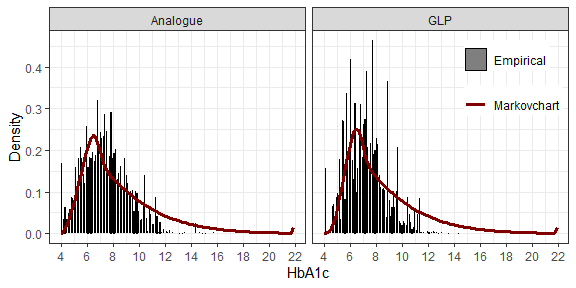The figure shows empirical and theoretical stationary distributions. We can assess that the stationary distribution fits the data at an acceptable level. This is actually a very beneficial result, as the main focus of the function is cost estimation which is based on the stationary distribution. Now that we have assured that there are no anomalies in the stationary distribution estimation, let us check the expected costs and cost standard deviations. The costs statistics are shown below.  ### Empirical costs for comparison # ANALOGUE mean(RANDOMISED_DATA[grepl("ANALOGUE", RANDOMISED_DATA$THERAPY) &
!grepl("GLP", RANDOMISED_DATA$THERAPY),]$THERAPY_COST_EUR)/30 +
mean(RANDOMISED_DATA[grepl("ANALOGUE", RANDOMISED_DATA$THERAPY) & !grepl("GLP", RANDOMISED_DATA$THERAPY),]$COST_CUMU) + sampling_cost/mean(deltats[,2]) #>  3.15981 mean(RANDOMISED_DATA[grepl("ANALOGUE", RANDOMISED_DATA$THERAPY) &
!grepl("GLP", RANDOMISED_DATA$THERAPY),]$THERAPY_COST_EUR)/30
#>  2.323821
mean(RANDOMISED_DATA[grepl("ANALOGUE", RANDOMISED_DATA$THERAPY) & !grepl("GLP", RANDOMISED_DATA$THERAPY),]$COST_CUMU) #>  0.7982125 sampling_cost/mean(deltats[,2]) #>  0.03777634 sd(RANDOMISED_DATA[grepl("ANALOGUE", RANDOMISED_DATA$THERAPY) &
!grepl("GLP", RANDOMISED_DATA$THERAPY),]$THERAPY_COST_EUR/30 +
RANDOMISED_DATA[grepl("ANALOGUE", RANDOMISED_DATA$THERAPY) & !grepl("GLP", RANDOMISED_DATA$THERAPY),]$COST_CUMU + sampling_cost/mean(deltats[,2])) #>  3.872857 res_ANALOGUE #>$Results
#>    G-value Expected cost Total cost sd Cost sd due to process var.
#> 1 3.167946      3.167946      3.752907                   0.2614257
#>   2nd process moment 3rd process moment 4th process moment
#> 1           10.10423           32.46643           105.1605
#>
#> $Subcosts #> Sampling cost Repair cost OOC cost #> 1 0.03777651 2.388222 0.7419474 #> #>$Parameters
#>   Time between samplings (h) Critical value (k)
#> 1                     206.22                  3

# GLP
mean(RANDOMISED_DATA[grepl("GLP", RANDOMISED_DATA$THERAPY),]$THERAPY_COST_EUR)/30 +
mean(RANDOMISED_DATA[grepl("GLP", RANDOMISED_DATA$THERAPY),]$COST_CUMU) +
sampling_cost/mean(deltats[,2])
#>  2.35354

mean(RANDOMISED_DATA[grepl("GLP", RANDOMISED_DATA$THERAPY),]$THERAPY_COST_EUR)/30
#>  1.88242
mean(RANDOMISED_DATA[grepl("GLP", RANDOMISED_DATA$THERAPY),]$COST_CUMU)
#>  0.4333437
sampling_cost/mean(deltats[,2])
#>  0.03777634

sd(RANDOMISED_DATA[grepl("GLP", RANDOMISED_DATA$THERAPY),]$THERAPY_COST_EUR/30 +
RANDOMISED_DATA[grepl("GLP", RANDOMISED_DATA$THERAPY),]$COST_CUMU +
sampling_cost/mean(deltats[,2]))
#>  3.493658

res_GLP
#> $Results #> G-value Expected cost Total cost sd Cost sd due to process var. #> 1 2.77835 2.77835 3.708439 0.2987063 #> 2nd process moment 3rd process moment 4th process moment #> 1 7.808456 22.24445 64.38612 #> #>$Subcosts
#>   Sampling cost Repair cost  OOC cost
#> 1    0.03777651    2.004543 0.7360308
#>
#> $Parameters #> Time between samplings (h) Critical value (k) #> 1 206.22 3 One can see that the total expected cost per day is 3.17€ for the analogue and 2.78€ for the GLP group, with considerable standard deviation (>3.7€) in both cases. (Note that p=1, thus the $$G$$-value is simply the expected cost.) The main source of the costs is the therapy cost, as we have seen during the parameter estimation. We can also compare the results to the empirical data for additional goodness of fit evaluation. It can be seen that the estimates of the Markovchart function are quite close to the ones estimated directly from the empirical data. Naturally there is room for improvement in some cases. For example the difference in the event costs is quite clear between therapies from the empirical data, while the Markovchart estimates do not reflect this. This is mainly an assumption problem: it is assumed that event (i.e., OOC) costs solely depend on the states of the chain (i.e., HbA1c level), thus due to the similar stationary distributions there will not be much difference between the event costs either. This of course can be solved by separate event cost estimates in the patient groups but since the absolute difference is small it is not important in this case. The sampling costs are exactly the same, as the empirical mean time between samplings (estimated from the whole patient population) was used in all cases. Overall, we can say that both the stationary distributions and the costs emulates the empirical data at an acceptable level. This is useful, because it means that we have a good baseline model which allows scenario exploration (i.e., changing various parameters to check their effects on the costs). We will now use the vectorisation feature of the Markovchart function and explore how the expected daily cost changes with different days between samplings and critical HbA1c levels. The code is almost the same as before, only the value of h and k is changed to numeric vectors. parall <- list(cl=makeCluster(num_workers), forward=FALSE, loginfo=TRUE) resmtx_ANALOGUE <- Markovchart( h = seq(90, 365, by = (365 - 90) / 19), k = seq(0.5, 6, by = (6 - 0.5) / 19), statdist = stat_ANALOGUE, p = 1, constantr = TRUE, ooc_rep = 1, cs = sampling_cost, coparams = summary(mod.COST)$coef[ , 1],
crfun = crfun_ANALOGUE,
crparams = summary(mod.ANALOGUE)$coef[ , 1], vcoparams = summary(mod_var.COST)$coef[ , 1],
vcrfun = vcrfun_ANALOGUE,
vcrparams = summary(mod_var.ANALOGUE)$coef[ , 1], parallel_opt = parall ) parall <- list(cl=makeCluster(num_workers), forward=FALSE, loginfo=TRUE) resmtx_GLP <- Markovchart( h = seq(90, 365, by = (365 - 90) / 19), k = seq(0.5, 6, by = (6 - 0.5) / 19), statdist = stat_GLP, p = 1, constantr = TRUE, ooc_rep = 1, cs = sampling_cost, coparams = summary(mod.COST)$coef[ , 1],
crparams = summary(mod.GLP)$coef[ , 1], vcoparams = summary(mod_var.COST)$coef[ , 1],
vcrparams = summary(mod_var.GLP)\$coef[ , 1],
parallel_opt = parall
)

We can use the plot method to quickly assess the results. The contour plot for the analogue therapy patient group is presented in the figure below.

resmtx_ANALOGUE[ , 2] <- resmtx_ANALOGUE[ , 2] + 4
plot(x = resmtx_ANALOGUE, y = 'Expected \ndaily cost',
mid = '#ff9494', high = '#800000',
xlab = 'Days between samplings',
ylab = 'Critical HbA1c level')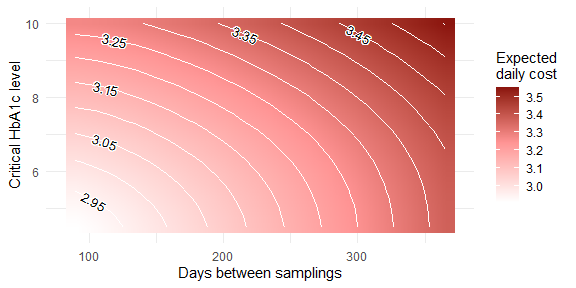Contour plot of expected costs (€) related to analogue therapy.

It can be seen that more frequent samplings and lower critical values entail less daily costs on average. The optimal parameters fall outside of the plotted area. This is due to the fact that lower parameter values are not viable. Namely, HbA1c measurements within 90 days of each other become redundant due to the slow variation and HbA1c values lower than 4% are actually indicating too low blood sugar. Nonetheless the results provide useful information about the relationship between the parameters, for example we can see that the expected daily costs is less sensitive on the differences between greater parameter values.

Since we have two different therapies it may be beneficial to compare them. The figure below conveys the same information as the one above, but for the GLP therapy.

resmtx_GLP[ , 2] <- resmtx_GLP[ , 2] + 4
plot(x = resmtx_GLP, y = 'Expected \ndaily cost',
mid = '#ff9494', high = '#800000',
xlab = 'Days between samplings',
ylab = 'Critical HbA1c level')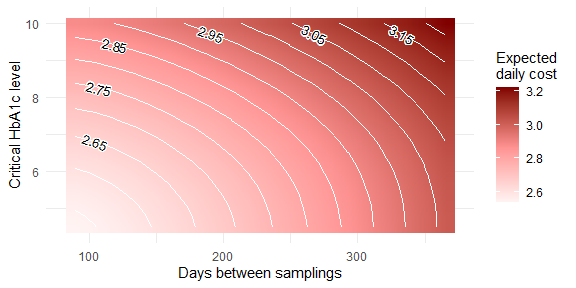It can be seen that the relationships between the variables are largely the same, however GLP is somewhat cheaper overall.